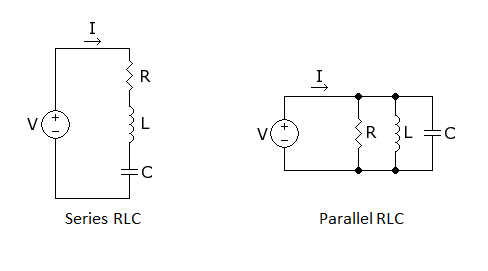RLC Circuit Resonant FrequencyThe formula for the resonant frequency of a series or parallel RLC circuit is:The important thing to remember is that it’s the same formula for either circuit.  Also, keep careful track of your decimal points.  Some of the questions have answers that are the same except for the decimal, (MHz vs. kHz).

Exam Questions

What is the resonant frequency of a series RLC circuit if R is 22 ohms, L is 50 microhenrys and C is 40 picofarads?

A. 44.72 MHz

B. 22.36 MHz

C. 3.56 MHz

D. 1.78 MHz

What is the resonant frequency of a series RLC circuit if R is 56 ohms, L is 40 microhenrys and C is 200 picofarads?

A. 3.76 MHz

B. 1.78 MHz

C. 11.18 MHz

D. 22.36 MHz

What is the resonant frequency of a parallel RLC circuit if R is 33 ohms, L is 50 microhenrys and C is 10 picofarads?

A. 23.5 MHz

B. 23.5 kHz

C. 7.12 kHz

D. 7.12 MHz

What is the resonant frequency of a parallel RLC circuit if R is 47 ohms, L is 25 microhenrys and C is 10 picofarads?

A. 10.1 MHz

B. 63.2 MHz

C. 10.1 kHz

D. 63.2 kHz